# Colleges with the highest SAT scores in Colorado

Top 10 colleges in Colorado with the highest SAT scores
Looking for the colleges with the highest SAT scores in Colorado? Well you're in luck! We've compiled a national college database and have created a list of the top 10 universities with the highest SAT scores in Colorado. These are the schools whose applicants had the highest average SAT scores in Colorado. And, since these tests are meant to determine academic prowess, they are arguably the schools with the most academically proficient students. You could even say these are the best colleges in Colorado. We also include each college's ACT scores and acceptance rate so that you can see where you would have the easiest or hardest time getting in. Read on to find out more.

The average SAT score for Colorado College is 1385.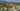The average SAT score of 1385 breaks down into:

• SAT math: 700

The average ACT score for Colorado College is 31 and their acceptance rate is 15%.

## Colorado School of Mines SAT scores

The average SAT score for Colorado School of Mines is 1360.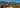The average SAT score of 1360 breaks down into:

• SAT math: 695

The average ACT score for Colorado School of Mines is 30 and their acceptance rate is 53.1%.

## University of Denver SAT scores

The average SAT score for University of Denver is 1270.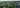The average SAT score of 1270 breaks down into:

• SAT math: 630

The average ACT score for University of Denver is 29 and their acceptance rate is 56.5%.

## University of Colorado Boulder SAT scores

The average SAT score for University of Colorado Boulder is 1252.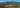The average SAT score of 1252 breaks down into:

• SAT math: 627

The average ACT score for University of Colorado Boulder is 28 and their acceptance rate is 81.5%.

## Naropa University SAT scores

The average SAT score for Naropa University is 1220.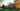The average SAT score of 1220 breaks down into:

• SAT math: 610

The average ACT score for Naropa University is 25 and their acceptance rate is 100%.

## Colorado State University SAT scores

The average SAT score for Colorado State University is 1180.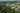The average SAT score of 1180 breaks down into:

• SAT math: 585

The average ACT score for Colorado State University is 26 and their acceptance rate is 81.4%.

## Regis University SAT scores

The average SAT score for Regis University is 1149.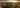The average SAT score of 1149 breaks down into:

• SAT math: 570

The average ACT score for Regis University is 24 and their acceptance rate is 60.4%.

The average SAT score for University of Colorado Colorado Springs is 1145.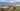The average SAT score of 1145 breaks down into:

• SAT math: 570

The average ACT score for University of Colorado Colorado Springs is 23 and their acceptance rate is 92.8%.

## University of Colorado Denver SAT scores

The average SAT score for University of Colorado Denver is 1120.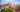The average SAT score of 1120 breaks down into:

• SAT math: 560

The average ACT score for University of Colorado Denver is 24 and their acceptance rate is 63.6%.

## Fort Lewis College SAT scores

The average SAT score for Fort Lewis College is 1113.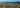The average SAT score of 1113 breaks down into:

• SAT math: 550